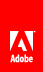# recalculate

Forces a specific set of scripts located on calculate events to execute. The specific events can be either pending calculate events or all calculate events.

#### Syntax

`Reference_Syntax.recalculate( BOOLEAN param )`

#### Parameters

 param A Boolean value indicating which calculation scripts should execute. true | 1 (JavaScript) or 1 (FormCalc) (default) All calculation scripts are re-executed. false | 0 (JavaScript) or 0 (FormCalc) Only pending calculation scripts should execute.

Empty

Model

Object

Form Model

form

template

XFA 2.1

#### JavaScript

`xfa.form.recalculate(1);`

#### FormCalc

`xfa.form.recalculate(1)`// Ethnio survey code removed